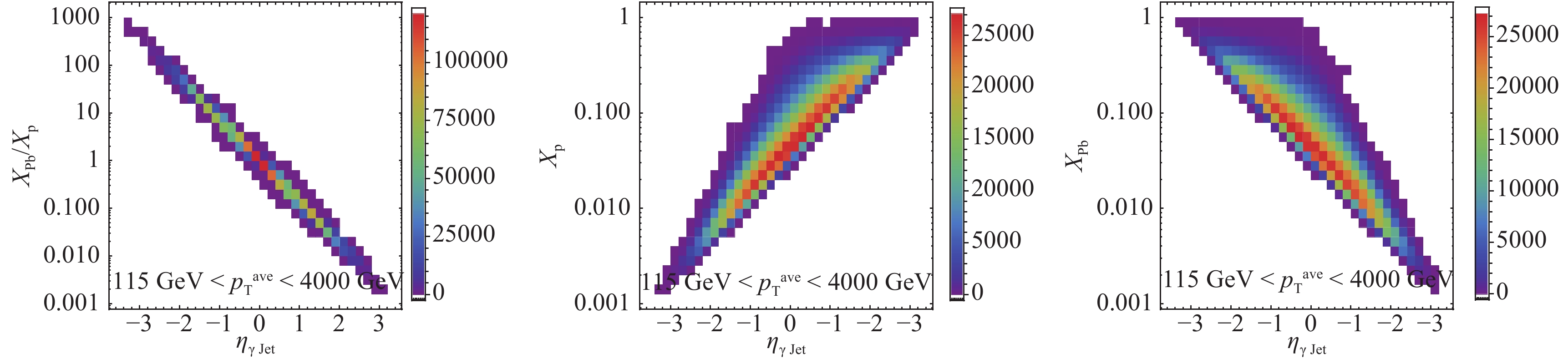# Probing cold nuclear matter effects with the productions of isolated-${\gamma}$and ${\gamma}$+jet in p+Pb collisions at ${\sqrt{{s}_{{NN}}}}=$8.16 TeV

• We investigate cold nuclear matter (CNM) effects on the productions of isolated prompt photons and $\gamma+$jet in proton-lead collisions at $\rm 8.16$TeV under next-to-leading order perturbative quantum chromodynamics calculations with four parameterizations for nuclear parton distribution functions (nPDFs), i.e., DSSZ, EPPS16, nCTEQ15, and nIMParton. Our theoretical calculations provide good descriptions of the pp baseline in the ATLAS collaboration and make predictions for future experimental results at $\rm p$+$\rm Pb$collisions. We calculate the dependence of the nuclear modification factor of isolated prompt photons on transverse momentum $p_{\rm T}^{\gamma}$and pseudo-rapidity $\eta^{\gamma}$at very forward and backward rapidity regions, and we demonstrate that the forward-to-backward yield asymmetries $Y_{\rm pPb}^{\rm asym}$as a function of $p_{\rm T}^{\gamma}$with different nPDF parameterizations have diverse behaviors. Furthermore, the nuclear modification factor of isolated-$\gamma+$jet $R_{\rm pPb}^{\gamma\rm Jet}$as a function of $\gamma+$jet's pseudo-rapidity $\eta_{\gamma \rm Jet}=\displaystyle\frac{1}{2}(\eta_{\gamma}+\eta_{\rm Jet})$at different average transverse momenta $p_{\rm T}^{\rm avg}=\displaystyle\frac{1}{2}(p_{\rm T}^{\gamma}+ p_{\rm T}^{\rm Jet})$has been discussed. This can facilitate a tomographic study of CNM effects with precise locations in a rather wide kinematic region by varying the transverse momenta and rapidities of both isolated photons and jets in p+A collisions.
••Get Citation
Guo-Yang Ma, Wei Dai and Ben-Wei Zhang. Probing cold nuclear matter effects with the productions of isolated- ${\gamma}$and ${\gamma}$+jet in p+Pb collisions at ${\sqrt{{s}_{{NN}}}}=$8.16 TeV[J]. Chinese Physics C. doi: 10.1088/1674-1137/43/4/044104
Guo-Yang Ma, Wei Dai and Ben-Wei Zhang. Probing cold nuclear matter effects with the productions of isolated- ${\gamma}$and ${\gamma}$+jet in p+Pb collisions at ${\sqrt{{s}_{{NN}}}}=$8.16 TeV[J]. Chinese Physics C.Milestone
Received: 2019-01-19
Article Metric

Article Views(101)
PDF Downloads(17)
Cited by(0)
Policy on re-use
To reuse of subscription content published by CPC, the users need to request permission from CPC, unless the content was published under an Open Access license which automatically permits that type of reuse.
###### 通讯作者: 陈斌, bchen63@163.com
• 1.

沈阳化工大学材料科学与工程学院 沈阳 110142

Title:
Email:

## Probing cold nuclear matter effects with the productions of isolated-${\gamma}$and ${\gamma}$+jet in p+Pb collisions at ${\sqrt{{s}_{{NN}}}}=$8.16 TeV

###### Corresponding author: Ben-Wei Zhang, bwzhang@mail.ccnu.edu.cn
• 1. Key Laboratory of Quark & Lepton Physics (MOE) and Institute of Particle Physics, Central China Normal University, Wuhan 430079, China
• 2. School of Mathematics and Physics, China University of Geosciences, Wuhan 430079, China

Abstract: We investigate cold nuclear matter (CNM) effects on the productions of isolated prompt photons and $\gamma+$jet in proton-lead collisions at $\rm 8.16$TeV under next-to-leading order perturbative quantum chromodynamics calculations with four parameterizations for nuclear parton distribution functions (nPDFs), i.e., DSSZ, EPPS16, nCTEQ15, and nIMParton. Our theoretical calculations provide good descriptions of the pp baseline in the ATLAS collaboration and make predictions for future experimental results at $\rm p$+$\rm Pb$collisions. We calculate the dependence of the nuclear modification factor of isolated prompt photons on transverse momentum $p_{\rm T}^{\gamma}$and pseudo-rapidity $\eta^{\gamma}$at very forward and backward rapidity regions, and we demonstrate that the forward-to-backward yield asymmetries $Y_{\rm pPb}^{\rm asym}$as a function of $p_{\rm T}^{\gamma}$with different nPDF parameterizations have diverse behaviors. Furthermore, the nuclear modification factor of isolated-$\gamma+$jet $R_{\rm pPb}^{\gamma\rm Jet}$as a function of $\gamma+$jet's pseudo-rapidity $\eta_{\gamma \rm Jet}=\displaystyle\frac{1}{2}(\eta_{\gamma}+\eta_{\rm Jet})$at different average transverse momenta $p_{\rm T}^{\rm avg}=\displaystyle\frac{1}{2}(p_{\rm T}^{\gamma}+ p_{\rm T}^{\rm Jet})$has been discussed. This can facilitate a tomographic study of CNM effects with precise locations in a rather wide kinematic region by varying the transverse momenta and rapidities of both isolated photons and jets in p+A collisions.

### HTML2.   Photon and photon+jet productions in P+P3.   Isolated photon in p+Pb collisions at very forward and backward rapidity4.   Isolated photon+jet in p+Pb
Reference (53)
PDF查看关注分享

Top

### 目录/DownLoad:  Full-Size Img  PowerPoint